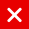# IOTA Tutorial

## NormalizedBundleHash

How the normalizedBundleHash is created, see: https://github.com/iotaledger/iota.li… Bundle.prototype.normalizedBundle = function(bundleHash)

The normalizedBundleHash is created by using the bundleHash. If you do not know what the bundleHash is, watch IOTA tutorial 15.

The normalizedBundleHash is used to create or validate a signature. This will be explained in detail in IOTA tutorial 17.

Divide the bundleHash in 3 parts, each part has 27 trytes: bundleHash = NBBKCKPFECRKCDIBKSTHZYZKSXF EUPTIJRK9FECFKPTTSWTLUWGIFS 9AHSDT9LASABRD9KDVFJ9GT9CKA
part0: NBBKCKPFECRKCDIBKSTHZYZKSXF
part1: EUPTIJRK9FECFKPTTSWTLUWGIFS
part2: 9AHSDT9LASABRD9KDVFJ9GT9CKA

For each part convert each tryte to its decimal value. part0: N=-13,B=2,B=2,K=11,.. part1: E=5,U=-6,P=-11,T=-7,.. part2: 9=0,A=1,H=8,S=-8,..

For each part calculate the sum. part0: sum = -13 + 2 + 2 + 11,.. = 45 part1: sum = 5 + -6 + -11 + -7,.. = 5 part2: sum = 0 + 1 + 8 + -8,.. = 42

In this tutorial we assume the bundleHash (=81 trytes) is already created.

If the sum is greater or equal than 0, the part has more “weight” on the right side. For each part the goal is to redistribute the “weight” evenly. This is done by taking the first tryte of the part and reduce its tryte value by 1 and calculate the sum.

Check if the sum is smaller than 0, if not keep reducing the tryte value. If the tryte value is -13, take the next tryte and do the same as before. Stop the process if the sum is smaller than 0.

If the sum is smaller than 0, the part has more “weight” on the left side. Again redistribute the “weight” evenly. This is done by taking the first tryte of the part and increase its tryte value by 1 and calculate the sum.

Check if the sum is greater or equal than 0, if not keep increasing the tryte value. If the tryte value is +13, take the next tryte and do the same as before. Stop the process if the sum is greater or equal than 0.

You can think of normalizing the bundleHash as balancing a seesaw, by manipulating its “weight” (=trytes) to reach a more equilibrium state.

If all 3 parts of the bundleHash are normalized, the normalizedBundleHash is created. It has the same number of trytes as the bundleHash (81 trytes). A normalizedBundleHash will not have any tryte value M. During the creation of the bundleHash, the normalizedBundleHash was also calculated to check if it contained tryte value M. If the normalizedBundleHash had value M, the obsoleteTag in the first transactionObject of the bundle was updated and the bundleHash was recalculated. This process was repeated until the normalizedBundleHash did not contained tryte value M.

• Question: Why not having tryte value M in the normalizedBundleHash?
• Answer: The normalizedBundleHash is used to create and validate the signature. During the creation of the signature (=signatureFragments), tryte value M will reveal a part of the private key.
• To recap: The bundle transactionObject addresses, values, obsoleteTags, timestamps, currentIndexes and lastIndexes are used to calculate the bundelHash. This bundelHash is added for each transactionObject in the bundle. To create or validate a signature the normalizedBundleHash is needed. The normalizedBundleHash is created by extracting the bundleHash from the transactionObject and normalizing the bundleHash. The normalizedBundleHash contains no tryte value M and the “weights” of the trytes are evenly distributed.
You might also like

This website uses cookies to improve your experience. We'll assume you're ok with this, but you can opt-out if you wish. AcceptRead More

Did you know?

Buy in without the stress of trying to time the market

when you setup a savings plan. If you haven’t started a plan yet, head to your Bitpanda account to get started!

Purchasing cryptocurrencies comes with a number of risks.
Never invest money that you can't afford to lose.You can automatically invest

in IOTA with Bitpanda Savings!